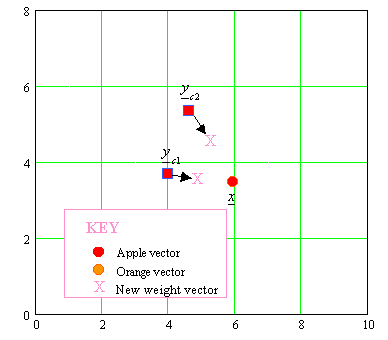# LEARNING VECTOR QUANTIZATION (LVQ)LVQ

• Recall that a Kohonen SOM is a clustering technique, which can be used to provide insight into the nature of data. We can transform this unsupervised neural network into a supervised LVQ neural network.
• The network architecture is just like a SOM, but without a topological structure.
• Each output neuron represents a known category (e.g. apple, pear, orange).
• Input vector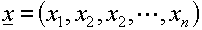• Weight vector for the jth output neuron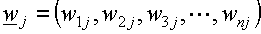•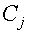= Category represented by the jth neuron.  This is pre-assigned.
• T = Correct category for input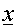• Define Euclidean distance between the input vector and the weight vector of the jth neuron as: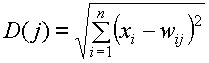LVQ Training Algorithm:

 STEP 0 Initialise weight vectors to the first m training vectors, where m is the number of different categories and set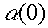. This weight initialization technique is presented here is only one of many different methods. 1 While stopping condition false, do steps 2 to 6. 2 For each training input vector x, do steps 3 to 4 3 Find J so that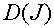is a minimum 4 Update the weights of the J neuron as follows: IF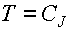THEN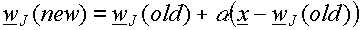(i.e. move the weight vector w toward the input vector x) IF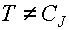THEN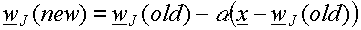(i.e. move w away from x) 5 Reduce learning rate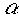6 Test stopping condition: This may be a fixed number of iterations or the learning rate reaching a sufficiently small value.

NOTE: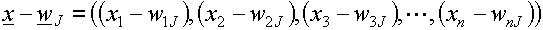EXAMPLE:  APPLES AND ORANGES

Suppose we measure the weight and height of three apples and three oranges.  The input vectorin this case would be x = (height, weight).  These input vectors are shown in the graph below.

• Using only two neurons in the output layer, let the initial weight vectors of the first and second neuron be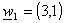and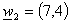respectively.

• Using a learning rate of 0.5, the graph below shows how the weight vectors of the two neurons change as the LVQ network is presented with the input vectors.  The order of presentation of the input vectors is
(1,3),  (3,4),  (6,1), ... (1, 6).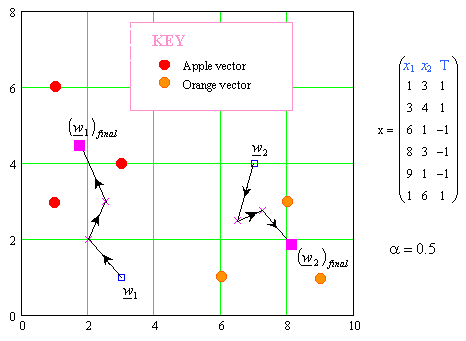EXAMPLE:  THREE CLASSES

Suppose there are three classes { red, blue and green}.  The applet animation below shows how an LVQ with two neurons per color, is able to adjust the weight vectors of its neurons so that they become typical red, blue and green reference or codebook  vectors.  As in the previous example, the input vector x has only two elements, which can then be shown on a 2D plot.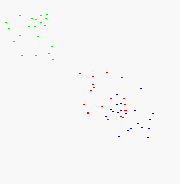EXAMPLE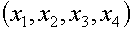T 1100 1 0001 2 0011 2 1000 1 0110 2

• Note there are only two different types of categories.
• We shall only use 2 neurons in the output layer, i.e. m = 2 as shown below.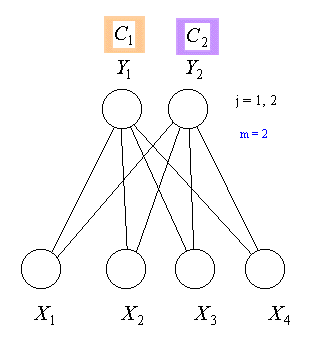TRAINING

Take the first two input vectors (since only 2 categories)  to set the weight vectors as follows: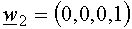Let us set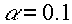For the input vector 0011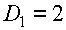,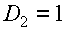Hence J = 2 since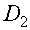is the minimum value

Now since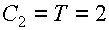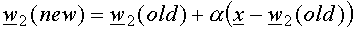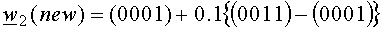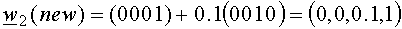For the input vector 1000

Find J = 1

Now since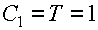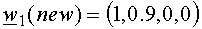For the input vector 0110

Find J = 1

Now since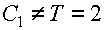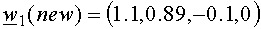STEP 5: Now reduce the learning rate

STEP 6: Test Stopping condition. : This may be a fixed number of iterations (excluding step 0 of course) or the learning rate reaching a sufficiently small value

##### Summary
• The weight vector for an output neuron is referred to as a reference or codebook vector for the category that the neuron represents.
• In the original LVQ algorithm, only the weight vector , or reference vector, which is closest to the input vector x is updated.
• After training, an LVQ network classifies an input vector by assigning it to the same category (or class) as the output neuron that its weight vector closest to the input vector.
• The improved variations on the LVQ algorithm [Kohonen 1990] are based on the idea that if the input vector is approximately the same distance from both the winner and the runner-up, then both of these weight vectors should be updated.

LVQ2 : First Improvement

Let= current input vector

Let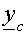= weight (or reference) vector that is closest toi.e the weight vector of the winner neuron in the output layer.

Let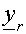= weight (or reference) vector that is the next closest toi.e. the weight vector of the runner-up neuron in the output layer.

Let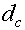= distance betweenandLet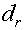= distance betweenandBoth vectors are updated if all of the following three conditions are satisfied:

1. The winning neuron and the runner-up represent different categories i.eandrepresent different categories.
2. The input vector belongs to the category as the runner-up i.ebelongs to the same category as.
3. The distances from the input vector to the winner and from the input vector to the runner-up are approximately equal. The specific condition is as follows:
 Update weights of the both winner and runner-up if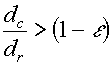AND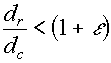where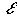depends on the number of training samples. For example for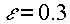the condition are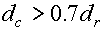ANDIf the above three conditions are satisfied, then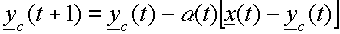i.e move weight vector away from input vector.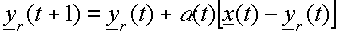i.e move weight vector towards from input vector.

EXAMPLEAPPLES AND ORANGES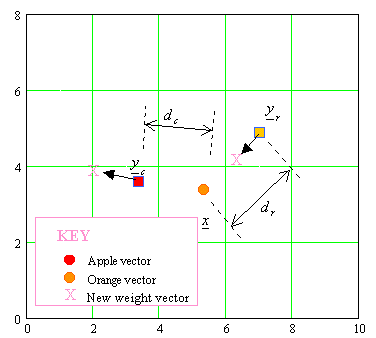LVQ2.1 : Second Improvement

Consider the two closest reference vectors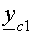and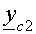The requirement for updating these vectors is that one of them belongs to the correct class (for the current input vector) and the other does not belong to the same class as• Note that we do not care whetheris closer toor to• Again it is also required thatfall in the "window" in order for an update to occur as follows: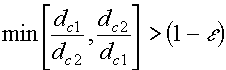AND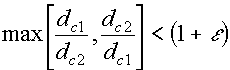If these conditions are met, and supposebelongs to the correct class, the reference vector that belongs to the same class asis updated using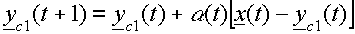i.e move weight vector towards from input vector.

and the reference vector that does not belong to the same class asis updated according to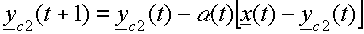i.e move weight vector away from input vector.

EXAMPLEAPPLES AND ORANGES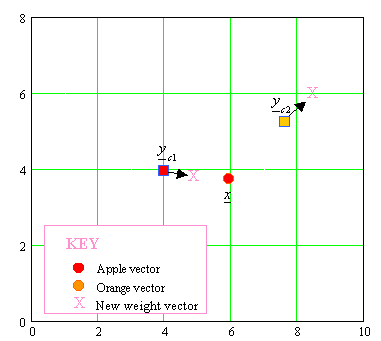LVQ3 : Third Improvement

Allow the two closest vectors to learn if the following condition is met: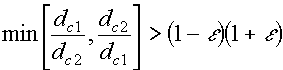where a typical value foris 0.2.
• If one of the two closest vectorsbelongs to the same class as the input vector, and the other vectorbelongs to a different class, the weight updates are as for LVQ2.1
• But ifandbelong to the same class, the weight updates arefor bothand.  The learning rate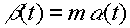, where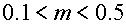.
• This modification to the learning process ensures that the weights (codebook vectors) continue to approximate the class distributions and prevents the codebook vectors from moving away from their optimal placement if learning continues.

EXAMPLEAPPLES AND ORANGES